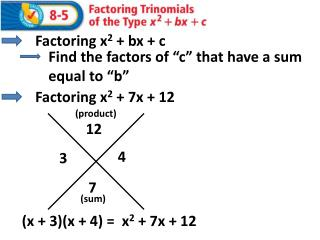# Factoring x 2 + 7x + 12 - PowerPoint PPT PresentationDownload PresentationFactoring x 2 + 7x + 12

Factoring x 2 + 7x + 12Download Presentation## Factoring x 2 + 7x + 12

- - - - - - - - - - - - - - - - - - - - - - - - - - - E N D - - - - - - - - - - - - - - - - - - - - - - - - - - -
##### Presentation Transcript

1. Factoring x2 + bx + c Find the factors of “c” that have a sum equal to “b” Factoring x2 + 7x + 12 (product) 12 4 3 7 (sum) (x + 3)(x + 4) = x2 + 7x + 12

2. Factoring x2 - 9x + 14 (product) 14 -7 -2 -9 (sum) (x - 2)(x - 7) = x2 - 9x + 14

3. Factoring x2 + 7x - 18 (product) -18 9 -2 7 (sum) (x - 2)(x + 9) = x2 + 7x - 18

4. Factoring x2 - 7x - 18 (product) -18 -9 2 -7 (sum) (x + 2)(x - 9) = x2 - 7x - 18

5. Factoring x2– 13xy + 42y2 (product) 42 -7 -6 -13 (sum) (x – 6y)(x – 7y) = x2– 13xy + 42y2